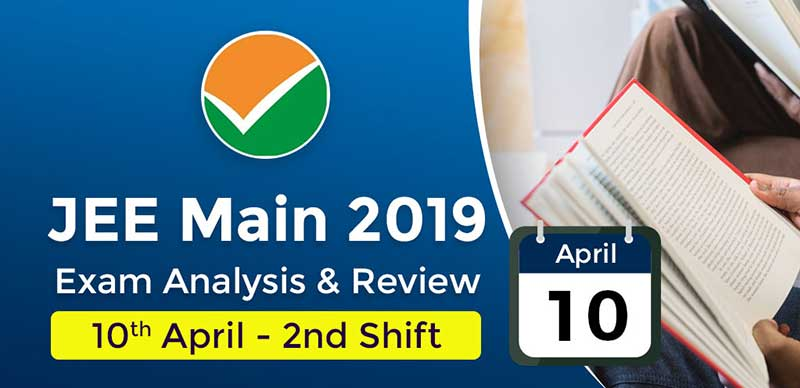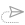### JEE Main 2019: Exam Analysis For Paper 1 (10th April - 2nd Shift)

The 2nd shift of JEE Main paper 1 has now ended for April 10, 2019. The analysis and reaction of students are provided here on this page.

Overall the paper in the 2nd shift was from difficult to moderate level as per candiadtes.

As per students physics again was difficult while mathematics was of moderate level and chemistry was the easiest section.

The Exam analysis is important to estimate the performance in the exam.

JEE Main 2019 Paper 1: Exam Format

 Subject No of Questions Marks Mathematics 30 120 Chemistry 30 120 Physics 30 120 Total 90 360

Analysis for Physics:

The physics paper was the toughest of all three and had equal weightage from all section.

Qs.1: What is the principle of Chromatography?

Qs.2: Light of wavelength 260nm falls on a metal plate whose threshold wavelength is 380 nm. Find the maximum kinetic energy of ejected electrons

Ans : 1.5 eV

Qs.3: Decay constants of two nuclei A and B are 10λ and λ respectively. Initially, the number of nuclei of A and B are same. Find the time at which ratio of nuclei of A and B is 1/E

Ans : 1/9λ

Qs.4: An ideal stepdown transformer turns in has 300 turns in primary coil and 150 turns in secondary coil. If the output power is 2.2 kW and current in primary coil is 10A. Then find the input volatge and current in secondary coil.

Ans : 110 V and 20 A

Qs.5: A particle with mass 2 kg collide with a another mass m elastically. And it moves in the same direction after collision with (1/4)th of its initial velocity, what will be the mass of other body

Ans : 1.2 kg

Qs.6: If the wavelength of a hydrogen spectrum in Balmer series from n = 3 to n = 2 is 660 nm. Find the wavelength if it makes a transition from n = 4 to n = 2

Ans : λ1/λ2=27/20

Qs.7: Two disc of moment of inertia I and I/2 about their respective axes (normal to the disc and passing through the center), and rotating with angular speed ω and ω/2 are brought together into contact with their axis of rotation coincident. After some time both the disc starts to rotate with a common velocity

Ans : 5ω/6

Qs.8: The surface mass density of a disc is given by σ = kr², where K is positive constant and 'r' is the distance from the center of the disc. What will be the moment of inertia of this disc? (The radius of disc is R and its total mass is M)

Ans : 2/3 MR²

Qs.9: Mass of a bullet was given it ht he box which was hung, Find the speed of the bullet?

Analysis for Chemistry:

talking about chemistry it was the easiest section with equal weightage to organic and inorganic. Organic chemistry was very easy as reported by a student.

Qs.1: Name the alloy which is used in making air craft?

Ans : Al-Mg

Qs.2: What is the Electrophilic aromatic substitution reactivity order?

Qs.3: What is the reactivity order of SN1 reactions?

Qs.4: Pair of Isoelectronic compounds is: a) Li+, Na+, F-, O2- b) Li+, Na3-, F-, O2- c) Li+, F-, N3-, Na+ d) N3-, O2-, F-, Na+

Ans : N3-, O2-, F-, Na+

Qs.5: Correct order of Catenation tendency is? a) C>Si>Ge≈ b) C>Ge≈Si>Sn c) C>Si≈Gn>Sn d) Sn>Ge>Si>C

Ans : C>Si>Ge≈

Qs.6: What should be ΔH & ΔS for process to be spontaneous reaction at all atmosphere a) ΔH>0; ΔS>0 b) ΔH0 c) Δ0, Δ

Ans : ΔH0

Qs.7: If 1/n part of chain is hanging then the work done to pull up the whole chain

Qs.8: Which is a condensation polymer?

Ans : Nylon 6,6

Analysis for Mathematics:

Mathematics was moderate level.

Qs.1: There were two points given on the graph P and Q on the intersection of two circles. Find the value of K

Qs.2: Range of x is given between (0,π), (0,π/2). Find the integral value of x in the given equation

Qs.3: The area bounded by curve |x+y| ≤ 2 and |x-2| ≤ 2 is

Ans : 16

Qs.4: The slope and area of region bounded by the curve |x=y| ≤ 2 and |x-y| ≤ 2 are

Ans : Square, 8 units

Qs.5: Let y=y(x) be a solution of differential equation dy/dx = (tanx-y)sec²x. If y(0) = 0, then y(-11/4) equals to

Ans : e-2

Qs.6: A line y=x touches a circle at point (1,1) which also passes through (1,-3). The radius of rhe circle is

Ans : 2√2

Qs.7: A line y=x touches a circle at point (1,1) which also passes through (1,-3). The radius of rhe circle is

Ans : 2√2

Student Reaction:

• Sequence and series question in mathematics also from coordinate geometry.
• 9-10 questions from organic chemistry.
• Question from Ionization enthalpy in chemistry.
• Numerical on Young’s double slit experiment question in ]physics.
• Questions from Both 11th and 12th were equally distributed.
• Algebra in mathematics held weightage.
• Maths was comparatively easier.
• More weightage was given to calculus in mathematics.
• Physics was the toughest section again.
• Almost all the topics were covered in physics
• Questions from Electrostatics were less
• Many questions from EM waves, although easy questions from semiconductor.
• Vector and 3 D in Maths.
• Chemistry was yet again the easiest
• Inorganic chemistry holds more weightage.20 September, 2021, 3:42 pm18 September, 2021, 10:34 am17 September, 2021, 10:55 am##### JEE Advanced 2021: Supreme Court Gives Permission To Students to Appeal JEE Board for 3rd Attempt

16 September, 2021, 11:40 am##### JEE Advanced Objective Physics 2021 : Topic-Wise Physics Quick Revision Notes With MCQs, NATs & Their Solutions

16 September, 2021, 11:35 am##### JEE Advanced 2021 : Registration Begins For Foreign Nationals, Indians Must Wait Till JEE Main Result

15 September, 2021, 11:40 amWe are providing something unique, useful and most importantly fun. By giving students a tool to find instant solutions to their doubts, we’re trying to make every student self-sufficient in practicing & completing their homework

### COMPANY×